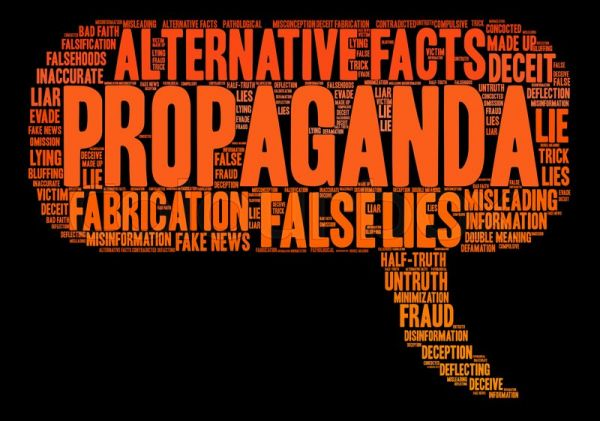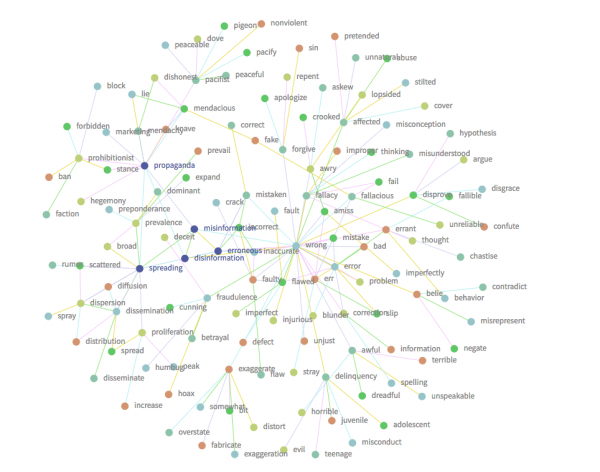# Datathon-HackNews-Solution-indianhunters

News is the lifeline of the human society , it underlines all the important events and influences public opinion like no other tool , but with the recent advent of electronic media and the sheer amount of new being churned out and the current political climate it’s hard to figure out what’s genuine news and what’s propaganda , this is where intelligent systems which can classify news articles , text fragments as propagandistic or non-propagandistic comes into play , this Datathon is focussed on developing such a system using various algorithms and methods to predict such a scenario the levels of challenges are:

A System that is able to classify a news article whether it is propaganda or not.
A System that is able to classify whether a sentence in a article is propaganda or not.
A System that is intelligently able to classify the propaganda technique used in the new piece.

0

# News Classification : Real or Fake

Team: Abhineet Singh, N.P. Ganesh, Nandan Gowda, Pooja Mohan, Rithvik Vorkady

##News Classification is done on various level with increasing level of complexity and different objectives as mentioned below:

• Propaganda detection at the article level (PAL). This is the easiest task, albeit not easy in absolute terms. It is a classical supervised document classification problem. You are given a set of news articles, and you have to classify each article in one of two possible classes: “propagandistic article” vs. “non-propagandistic article.”
• Propaganda detection at the sentence level (PSL). This is another classification task, but of different granularity. The objective is to classify each sentence in a news article as either “sentence that contains propaganda” or “sentence that does not contain propaganda.”
• Propaganda type recognition (PTR). This task is similar to the task of Named Entity Recognition, but applied in the propaganda detection setting. The goal is to detect the occurrences and to correctly assign the type of propaganda to text fragments.

## Methodology Used:

We found the F1 score first – ‘The computation of the F1 score will be done as follows: First, we compute the precision P as the ratio of the number of true positives (documents/sentences that the system has labeled as propagandistic and they are indeed such) and the total number of documents/sentences that the system has classified as propagandistic. Second, we compute the recall R as the ratio of the number of true positives and the number of all documents that are indeed propaganda. Then, F1 = 2PR / (P + R). This measure takes into consideration the class imbalance in the testing dataset.’. this was all computed using Python.

### PACKAGES USED:

We used the scikit learn package of the Python which has the Countvectorizer, TfidfVectorizer, HashingVectorizer

COUNTVECTORIZER():

Convert a collection of text documents to a matrix of token counts

This implementation produces a sparse representation of the counts using scipy.sparse.coo_matrix.

If you do not provide an a-priori dictionary and you do not use an analyzer that does some kind of feature selection then the number of features will be equal to the vocabulary size found by analyzing the data.

TFIDFVECTORIZER():

Convert a collection of raw documents to a matrix of TF-IDF features.

Equivalent to CountVectorizer followed by TfidfTransformer(Apply Term Frequency Inverse Document Frequency normalization to a sparse matrix of occurrence counts.)

HASHINGVECTORIZER():

Convert a collection of text documents to a matrix of token occurrences

It turns a collection of text documents into a scipy.sparse matrix holding token occurrence counts (or binary occurrence information), possibly normalized as token frequencies if norm=’l1′ or projected on the euclidean unit sphere if norm=’l2′.

This text vectorizer implementation uses the hashing trick to find the token string name to feature integer index mapping.

• it is very low memory scalable to large datasets as there is no need to store a vocabulary dictionary in memory
• it is fast to pickle and un-pickle as it holds no state besides the constructor parameters
• it can be used in a streaming (partial fit) or parallel pipeline as there is no state computed during fit.
LIBRARIES USED:
import pandas as pd
from sklearn.model_selection import train_test_split
from sklearn.feature_extraction.text import CountVectorizer
from sklearn.feature_extraction.text import TfidfVectorizer
from sklearn.feature_extraction.text import HashingVectorizer
from sklearn import metrics
from sklearn.naive_bayes import MultinomialNB
from sklearn.linear_model import LogisticRegression
from sklearn.linear_model import PassiveAggressiveClassifier
from sklearn.ensemble import RandomForestClassifier
from sklearn.tree import DecisionTreeClassifier
from sklearn.metrics import confusion_matrix
from sklearn.neighbors import KNeighborsClassifier
from sklearn.svm import SVC
from sklearn.neural_network import MLPClassifier

with the plethora of varying opinion in public discourse right now , news has been playing a critical role in informing people with the current developments, its more critical than ever that the news that is being circulated is known to be accurate and closer tot he truth rather than a propaganda piece.

our aim is to detect the news pieces at article level, then at the sentence level and then classify into the propaganda type.

we will be using supervised machine learning techniques to build a model that would be able to identify and flag the false news propaganda.

Data Understanding and Prepping:

First step in any sort of Data Classification is to first convert it into a workable format the development data is not in the “CSV” format , The news text, news number and whether it is a propaganda news or non-propaganda news are mentioned side-by-side and all are in a jumbled format. It needs to be modified into a “CSV” file so that we can use the machine learning algorithm to predict the type of news

Train data is in textual format with 35993 propagandistic article with article id and label of the article (“propaganda”, “non-propaganda”) This train data set has been converted into a .csv file:

1) The first column contains the title and the contents of the article

2) The second column is the article id;

3) The third column is the label of the article

Data Cleansing

The next step of the analysis is to clean the data and make it ready for using it in the model – which s is done using the various built in functions present in Python.

The NA values are removed, the unnamed columns are dropped, the columns are arranged in the ordered format, and index is set.

Modelling:

The F1 score and accuracy level were calculated using the following ML classifier algorithms:

• Naïve Bayes Multinomial Classifier Model
• Passive Aggressive Classifier Model
• Decision Tree Classifier Model
• Random Forest Classifier Model
• k-NN Classifier Model
• Logistic Model
• Support Vector Machine
• XGBoost Model
• Multiple Layer Perceptron Classification Model (Feed Forward Neural Network)

Text data requires special preparation before you can start using it for modelling.The text must be parsed to remove words, called tokenization. Then the words need to be encoded as integers or floating point values for use as input to a machine learning algorithm, called feature extraction (or vectorization). The scikit-learn library offers easy-to-use tools to perform both tokenization and feature extraction of your text data.

The data set has been split as training data and testing data with 30% of the data being assigned to the test dataset and the remaining 70% of the data as training data. This is done using the ‘train_test_split’ function of sklearn library (as shown below)

## INFERENCE:

As per the results obtained it is determined that the PassiveAgressiveClassifier ML Classifier Algorithm provided the best fit for the data while using the TF-IDF vecotrizer as the accuracy and F1 score obtained were the highest in this setup.

NOTE: FOR TRAIN DATA THE RANDOM STATE WE USED IS 42  ,AS RANDOM STATE NEEDS TO HAVE FIXED INTEGER VALUE OTHERWISE IT RANDOMLY TAKES VALUES.

## Propaganda detection at the Sentence level (PSL):

The objective is to classify each sentence in a news article as either “sentence that contains propaganda” or “sentence that does not contain propaganda.”

 Count Vectorization HashingVectorizer TfidfVectorizer Acccuracy(%) F1(Absolute) Acccuracy(%) F1(Absolute) Acccuracy(%) F1(Absolute) I. Naïve Bayes Multinomial Classifier Model 71.50 0.495 – – 73.30 0.117 II. Passive Aggressive Classifier Model 71.21 0.442 73.10 0.460 71.70 0.462 III. Decision Tree Classifier Model 69.91 0.462 70.10 0.424 69.70 0.422 IV. Random Forest Classifier Model 72.51 0.402 73.50 0.265 73.50 0.313 V. k-NN Classifier Model 71.82 0.128 71.70 0.503 72.00 0.052 VI. Logistic Model 75.42 0.451 74.10 0.250 74.12 0.242 VII. Support Vector Machine 71.94 NaN 71.90 NaN 71.90 NaN VIII. XGBoost Model 73.18 0.250 72.90 0.239 73.00 0.255 IX.MLP Classification(Feed Forward Neural Network)(5) 70.34 0.444 70.30 0.448 70.60 0.456 IX.MLP Classification(Feed Forward Neural Network)(5,5) 70.00 0.442 70.30 0.482 69.80 0.495 IX.MLP Classification(Feed Forward Neural Network)(5,5,5) 0.692 0.446 70.10 0.442 70.15 0.448

## INFERENCE:

As per the results obtained it is determined that the K-NN ML Classifier Algorithm provided the best fit for the data while using the Hashing vectorizer as the accuracy and F1 score obtained were the highest in this setup.

NOTE: FOR TRAIN DATA THE RANDOM STATE WE USED IS 42  ,AS RANDOM STATE NEEDS TO HAVE FIXED INTEGER VALUE OTHERWISE IT RANDOMLY TAKES VALUES.

### OUTPUT: